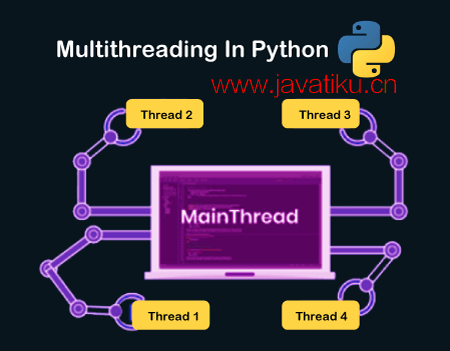# Tkinter 教程-Python 3中的多线程## Python 3中的多线程

### 使用Python进行多线程的优势

1. 它保证了计算机系统资源的有效使用。
2. 具有多个线程的应用程序响应更快。
3. 它更具成本效益，因为它与子线程（子线程）共享资源和状态。
4. 由于亲密度，多处理器架构更加有效。
5. 通过同时运行多个线程，可以节省时间。
6. 系统不需要大量内存来存储多个线程。

1. 线程模块
2. 线程模块

### 线程模块

``thread.start_new_thread ( function_name, args[, kwargs] )  ``

``````import thread # import the thread module
import time # import time module

def cal_sqre(num): # define the cal_sqre function
print(" Calculate the square root of the given number")
for n in num:
time.sleep(0.3) # at each iteration it waits for 0.3 time
print(' Square is : ', n * n)

def cal_cube(num): # define the cal_cube() function
print(" Calculate the cube of  the given number")
for n in num:
time.sleep(0.3) # at each iteration it waits for 0.3 time
print(" Cube is : ", n * n *n)

arr = [4, 5, 6, 7, 2] # given array

t1 = time.time() # get total time to execute the functions
cal_sqre(arr) # call cal_sqre() function
cal_cube(arr) # call cal_cube() function

print(" Total time taken by threads is :", time.time() - t1) # print the total time  ``````

``````Calculate the square root of the given number
Square is:  16
Square is:  25
Square is:  36
Square is:  49
Square is:  4
Calculate the cube of the given number
Cube is:  64
Cube is:  125
Cube is:  216
Cube is:  343
Cube is:  8
Total time taken by threads is: 3.005793809890747``````

### 线程模块

start()start()方法用于启动线程的活动。它只为每个线程对象调用一次，以便线程的执行可以开始。
run()run()方法用于定义线程的活动，并且可以由扩展线程类的类重写。
join()join()方法用于阻止其他代码的执行，直到线程终止为止。

1. 导入线程模块

``import threading  ``

2. 声明线程参数： 它包含目标函数、参数和 kwargs 作为 Thread() 类中的参数。

• 目标： 它定义了由线程执行的函数名称。
• 参数： 它定义了传递给目标函数名称的参数。

``````import threading
def print_hello(n):
print("Hello, how old are you ", n)

3. 启动新线程： 要在Python多线程中启动线程，请调用线程类的对象。 start() 方法可以为每个线程对象调用一次；否则，它将引发异常错误。

``````t1.start()
t2.start() ``````

4. Join方法： join() 方法用于阻止主线程的执行，并等待线程对象的完整执行。当线程对象完成时，它开始执行Python中的主线程。

Joinmethod.py

``````import threading
def print_hello(n):
Print("Hello, how old are you? ", n)
T1.start()
T1.join()
Print("Thank you") ``````

``````Hello, how old are you? 20
Thank you``````

#### 注意：如果不使用join()方法，解释器可以执行Python程序中的任何打印语句。通常情况下，它会执行第一个打印语句，因为解释器会从程序的开头执行代码行。

5. 在Python中同步线程

``````import time # import time module
def cal_sqre(num): # define a square calculating function
print(" Calculate the square root of the given number")
for n in num: # Use for loop
time.sleep(0.3) # at each iteration it waits for 0.3 time
print(' Square is : ', n * n)

def cal_cube(num): # define a cube calculating function
print(" Calculate the cube of  the given number")
for n in num: # for loop
time.sleep(0.3) # at each iteration it waits for 0.3 time
print(" Cube is : ", n * n *n)

ar = [4, 5, 6, 7, 2] # given array

t = time.time() # get total time to execute the functions
#cal_cube(ar)
#cal_sqre(ar)
th1.start()
th2.start()
th1.join()
th2.join()
print(" Total time taking by threads is :", time.time() - t) # print the total time
print(" Again executing the main thread")

``````Calculate the square root of the given number
Calculate the cube of the given number
Square is:  16
Cube is:  64
Square is:  25
Cube is:  125
Square is:  36
Cube is:  216
Square is:  49
Cube is:  343
Square is:  4
Cube is:  8
Total time taken by threads is: 1.5140972137451172